Email us to get an instant 20% discount on highly effective K-12 Math & English kwizNET Programs!

#### Online Quiz (WorksheetABCD)

Questions Per Quiz = 2 4 6 8 10

### High School Mathematics - 28.12 Construction of a Tangent to a Circle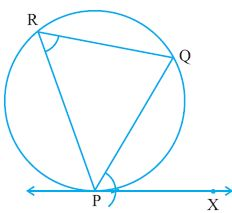Step 1: Draw any chord PQ of the circle through P as shown. Step 2: Take any point R on the major arc PQ. Join PR and QR. Step 3: COnstruct angle OPX equal to angle PRQ. PX is the required tangent at P. Steps to construct a tangent to a circle from an external point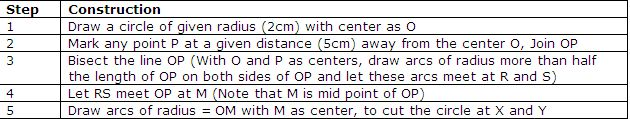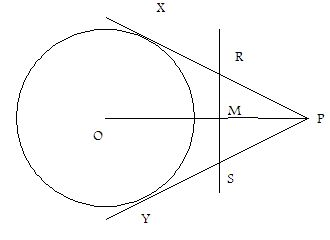Construction of Direct common tangents to 2 circles of equal radii whose centers are at a given distance from each other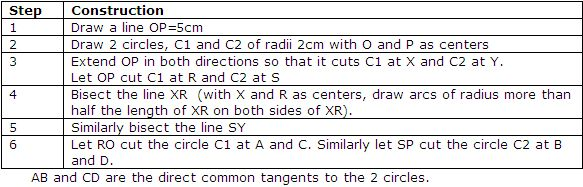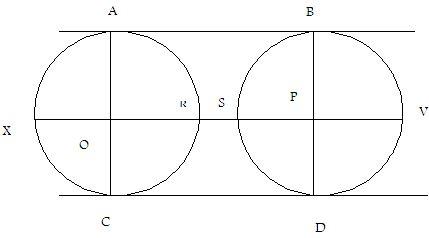Construction of Direct common tangents to 2 circles of different radii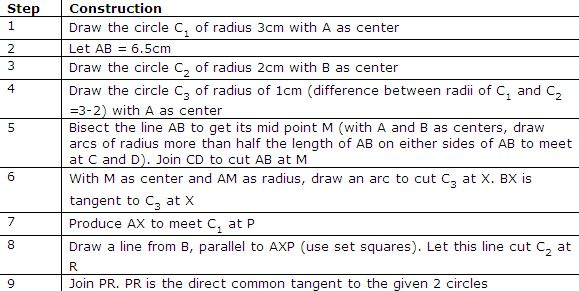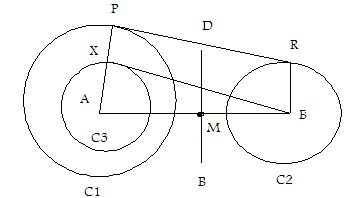Construction of tranverse tangents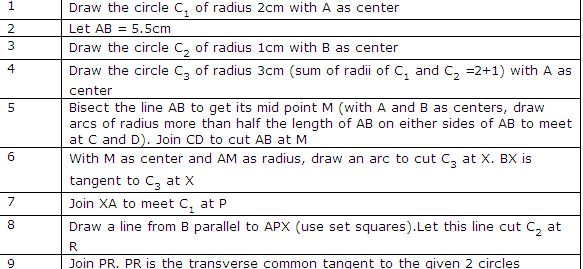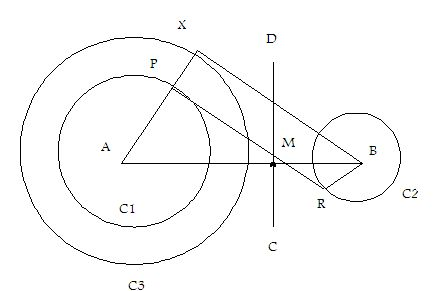Directions: Construct tangents to the following.